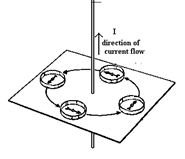# CBSE 12TH PHYSICS - Online Test

Q1. Work done by a uniform electric field E in moving a charge q a distance d from a to b is
Explaination / Solution:

Force and the work done
Q2. What is the percentage of the mass of an atom concentrated in the nucleus?
Explaination / Solution:

A proton and a neutron have roughly 2000 times the mass of an electron. The numbers of an electron in a stable atom (not isotope) is equal to the electrons. So for most atoms, the nucleus will hold roughly 4000 times the mass of its electron cloud. Thus,
Q3. Light rays from a point object
Explaination / Solution:
No Explaination.

Q4. A wire is perpendicular to the plane of the paper. A ring of compass needles surrounds the wire in the plane of the paper with center of ring being the center of the wire .Initially there is no current in the wire. What happens after a steady dc current is established in the wire?
Explaination / Solution:

The current carrying wire has a magnetic field around it and the lines of force are in the form of concentric circles with their centers on the wire.Q5. Two cells of 1.25 V and 0.75 V are connected in series with anode of one connected to cathode of the other . The effective voltage will be
Explaination / Solution:

The cells support each other.
Q6. Is light a particle or a wave?
Explaination / Solution:

Light is an electro magnetic wave, it has dual nature and behave as both particle and wave

Q7. which of the following statements about earth's magnetism is correct
Explaination / Solution:
No Explaination.

Q8. Basic materials used in the present solid state electronic devices like diode, transistor, ICs, etc are
Explaination / Solution:

Semiconductors are materials that have electrical conductivity between conductors such as most metals and nonconductors or insulators like ceramics. Semiconductor material is used in the manufacturing of electrical components and used in electronic devices such as transistors and diodes. Semiconductors are the basic materials used in the present solid state electronic devices like diode, transistor, ICs etc

Q9. According to Maxwell’s equations
Explaination / Solution:
No Explaination.

Q10. electromagnetic induction i.e currents can be induced in coils (Select the best)
Explaination / Solution:

for electromagnetic induction magnet and coil both may move but relative motion between must be present